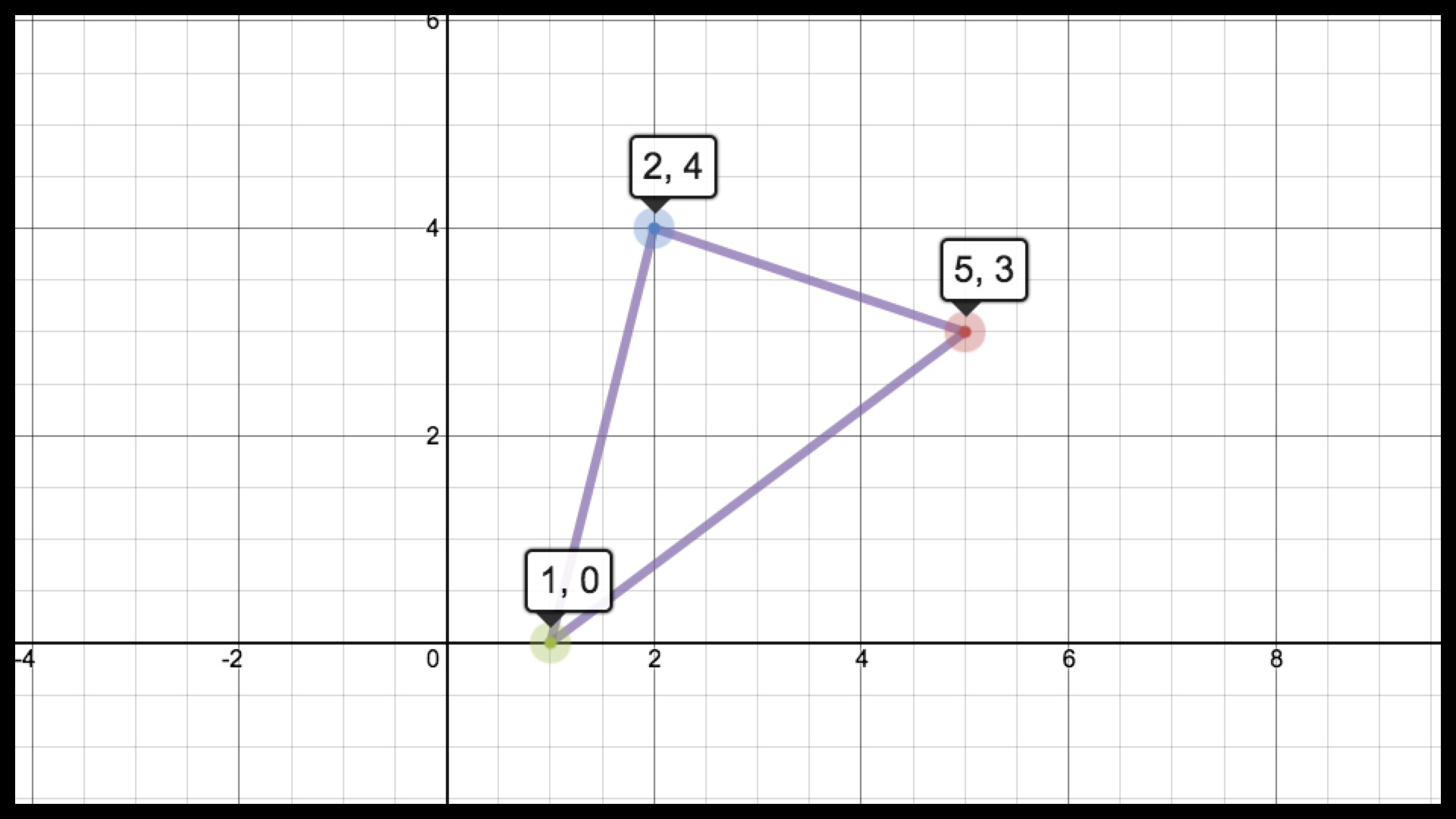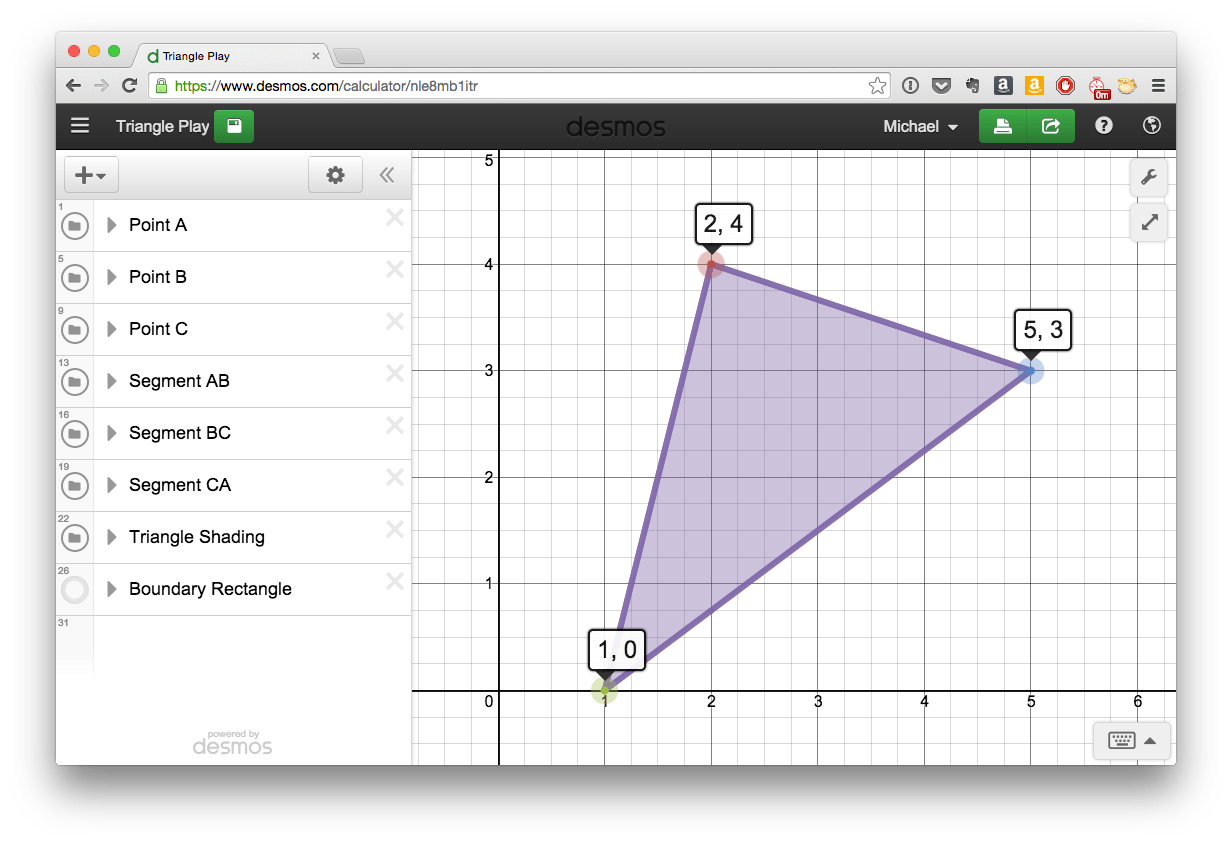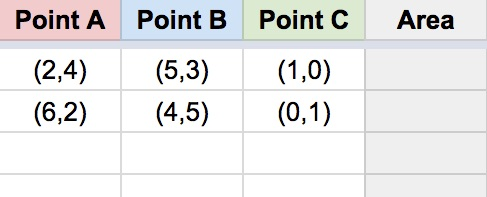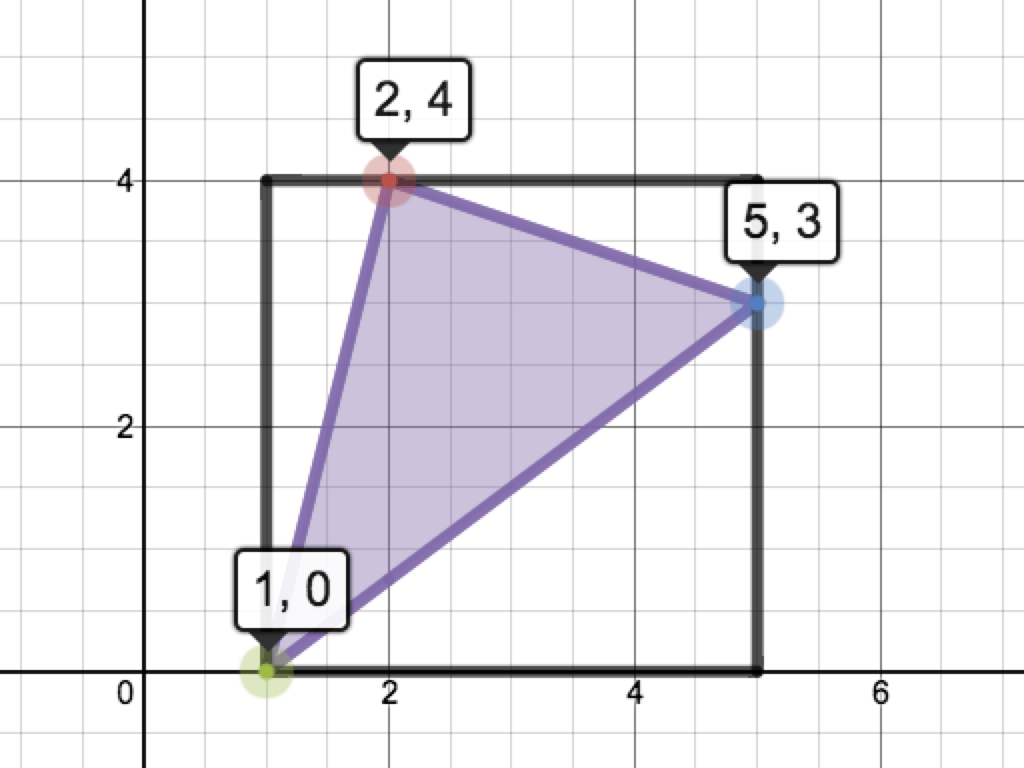# Area Boss

Develop your students’ area-finding abilities with this coordinate-plane-based triangle challenge:### Challenges

1. Use six different digits (0-9) to create three ordered pairs that determine a triangle. What is the largest such triangle you can build?

### Lesson Notes

Display the following image:Ask students to write down 2-3 things they notice. After a moment or two, ask volunteers to share one or more of their responses. Make a record of their observations. One of the key things to notice (at least for the sake of this task) is that the three ordered pairs consist of six different digits. If no student makes this observation, highlight this fact before moving on. While there is no natural motivation to use six different digits, doing so provides a nice upgrade to the overall difficulty of the task.

Next, display the following instructions:The link in the image above will direct students to the following Desmos graph, which is intended to help students visualize their approach to the problem (the three points are “movable”):Give students some time to explore the graph. As they begin to find triangles, have them write the three ordered pairs (along with the triangle’s area) on the board. I recommend organizing the results in a table, possible like this:Depending on your students’ familiarity with oblique triangles, they may struggle to find the area. Older high school students likely have several strategies at their disposal. (I’ll highlight these in a future update to the lesson.) However, this task is quite accessible to younger students, given the right approach. If working with students who lack trigonometry-based area-finding skills, consider displaying this image as a hint:To find the area of the triangle pictured above, find the area of the rectangle, then subtract the areas of the three right triangles that are inside the black rectangle, but outside the shaded region.

As the board fills up with more and more answers, challenge students to do two things:

• Confirm that the areas other students have submitted to the board are correct
• Search for a triangle larger than those already submitted

If a student or group believes they have found the triangle with the absolute greatest area, ask them to construct an argument about why no larger triangle is possible.

### Extensions

1. For other ideas on using this Desmos-based triangle applet, consider pursuing one of these Twitter-sourced extensions.

### Key Standards

• CCSS 6.G.A
Solve real-world and mathematical problems involving area, surface area, and volume.
• CCSS 7.G.B
Solve real-life and mathematical problems involving angle measure, area, surface area, and volume.

### Supporting Standards

• CCSS 5.G.A
Graph points on the coordinate plane to solve real-world and mathematical problems.

### More Lessons

Looking for more lessons? Click here.